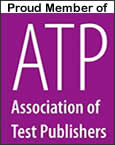# Math -- Intermediate Skills Test

Test Summary: An assessment aimed at measuring a candidate's proficiency regarding quickly performing basic mathematical computations such as addition, subtraction, multiplication and division, as well as calculating percentages, and converting fractions and decimals.

Test Specifications:
• Test Name: Math -- Intermediate Skills Test
• Job Level: Legal
• Test Publisher: SHL
• Uses: Assess a candidate's proficiency regarding quickly performing basic mathematical computations
• Estimated Testing Time: 6 minute time limit (70 questions)
• Test Format: Multiple Choice
Abilities, Knowledge & Skills Measured:
• Perform basic mathematical computations
Order This Assessment